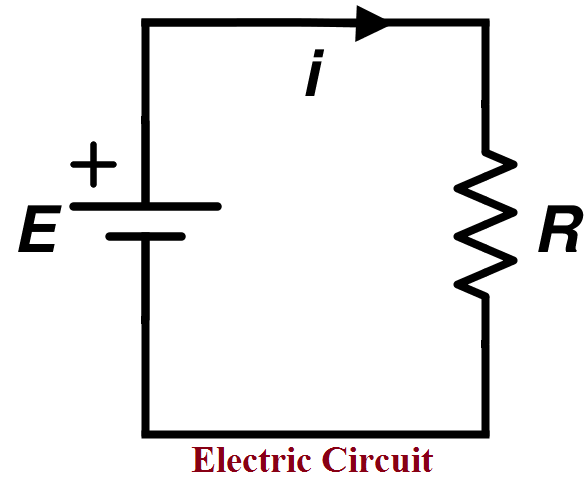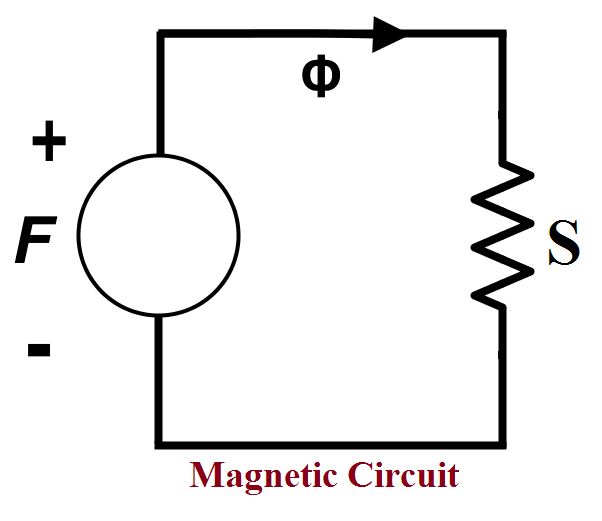# 14 Difference Between Electrical Circuit and Magnetic CircuitHello!

In the electromagnetic environment, electric and magnetic field studies are most important. Many similarities and dissimilarities find out in both fields.

Mostly, both fields have a different approach. So, you should know the difference between the Electrical field and the Magnetic field.

I am explaining more things through the difference between Electrical circuit and Magnetic Circuit. These concepts will help you understand both fields.

Let’s start the point by point…

### 14 Difference Between Electrical Circuit and Magnetic Circuit

Let’s start with the different points from both circuit,

Sr.No. Content Electrical Circuit Magnetic Circuit
1 Basic Difference In an electrical circuit, electric current flows through the path. In the magnetic circuit, magnetic flux flows through the path.
2 Definition

(Symbol, Unit & Formula)

The number of free electrons or electric charge particles move in the circuit is called ‘Electric Current’.

It denoted by ‘I’.

The S.I. unit is ‘A’ (Ampere)

The formula of Current,

`I=(EMF/R)=(V/R)`
The number of magnetic lines of the force passing through a magnet is called’Magnetic Flux’.

It denoted by‘ɸ’.

The S.I. unit is ‘Wb’ (Weber).

The formula of Flux,

`ɸ=(MMF/S)`
3 Circuit Role In this circuit, electric current flows from positive to negative polarities. In this circuit, magnetic flux flows from N-pole to S-pole.
4 Force

(Symbol & Unit)

The force is necessary to move the free electron in a particular direction and produce current. this force called ‘Electromotive Force’.

Its denoted by ‘EMF’.

EMF in ‘V’ (Volt).

The force is applied to the number of magnetic field lines passing through a surface. this force called ‘Magnetomotive Force’.

Its denoted by ‘MMF’.

MMF in ‘AT’ (Ampere-turn).

5 Property I

(Opposition Property)

The properties of the substance which oppose the flow of electric current are called ‘Resistance’.

Resistance denoted by ‘R’.

The S.I. unit is ‘Ω’ (Ohm).

The formula,

`R=(ρl/a)`

Where ρ is Resistivity.

The properties of the substance which oppose the flow of magnetic flux are called  ‘Reluctance’.

Reluctance denoted by ‘S’.

The S.I. unit is ‘AT/Wb’ (Ampere- turn/Weber).

The formula,

`S=(l/µa)`

Where µ is Permeability

6 Property II

(Flowing Property)

Conductance is the reciprocal of the resistance.

Its denoted by ‘G’.

The S.I. unit is ‘S’ (Siemens).

The Formula,

`G=(1/R)`
Permeance is the reciprocal of the reluctance.

Its denoted by ‘P’.

The S.I. unit is ‘Wb/AT’ or ‘H’(Weber/Ampere- turn or Henry).

The Formula,

`p=(1/S)`
7 Force

(Calculation Of Force)

Total EMF,

`Ʃ (EMF)=IR1+IR2+IR3+...`
Total MMF,

`Ʃ (MMF)=ɸS1+ɸS2+ɸS3+...`
8 Circuit’s Law According to Ohm’s Law,

`I=(E/R)=(EMF/R)`
According to Ohm’s Law,

`ɸ=(F/S)=(MMF/S)`
9 Circuit’s First Law According to Kirchhoff’s First Law,

`Ʃ I =0 at a node.`
According to Kirchhoff’s First Law,

`Ʃ ɸ=0 at a node.`
10 Circuit’s Second Law According to the Kirchhoff’s Second Law, for the closed circuit.

`Ʃ (IR)= Ʃ (EMF)`

According to the Kirchhoff’s Second Law, for the closed circuit.

`Ʃ (ɸS)= Ʃ (MMF)`

11 Density

(Symbol, Unit & Formula)

Current Density is the rate of the electric currents flowing per unit cross-sectional area of a material.

It is denoted by the ‘δ’.

The S.I. unit is A/m2
(Ampere/sq. meter).

The Formula,

` δ=(I/a)`
Flux Density is the rate of the magnetic fluxes flowing per unit cross-sectional area of a material.

It is denoted by the ‘B’.

The S.I. unit is Wb/m2 or T
(Weber/sq. meter or Tesla).

The Formula,

`B=(ɸ/a)`
12

Strength

(Symbol, Unit & Formula)

Electric Field Strength
is the Electromotive force per unit electric charge.It is known as ‘Electric Field Intensity’.It is denoted by the ‘E’.The S.I. unit is N/C or V/m
(Newton/coulomb or volt/meter).The Formula,

`E=(EMF/q)`
Magnetic Field Strength
is the Magnetomotive force per unit length.It is known as ‘Magnetic Field Strength’.It is denoted by the ‘H’.The S.I. unit is A/m
(Ampere/meter).The Formula,

`H=(MMF/l)`
13 Circuit Structure The electrical circuit can be closed or open circuit. The magnetic circuit is always a closed circuit.

14. Circuit Diagram:

• Electrical Circuit

You can see the following diagram, the resistance is connected in the electrical circuit. This resistance (R) is helped to limit the producing current (i) in the electrical circuit.• Magnetic Circuit:

The same working function has done. But in the magnetic circuit, we are used reluctance (S) to limit the generating magnetic flux (Φ). You can understand from the below figure.The phenomena of the interrelation of an electric field and the magnetic field are known as ‘Electromagnetism’. That we will see in the upcoming article.

#### Reference Links for More Understanding:

That’s all!

In this tutorial, I have covered many concepts and near about 14 dissimilarities/difference between the electrical circuit and magnetic circuit.

If you have any doubt or any point to include, feel free to discuss in the comment section.

Thank You!

If You Appreciate What I Do Here On DipsLab, You Should Consider:

DipsLab is the fastest growing and most trusted community site for Electrical and Electronics Engineers. All the published articles are available FREELY to all.

If you like what you are reading, please consider buying me a coffee ( or 2 ) as a token of appreciation.

This will help me to continue my services and pay the bills.I’m thankful for your never-ending support.

### 2 thoughts on “14 Difference Between Electrical Circuit and Magnetic Circuit”

1.Nice job. Thanks, I am always waiting for your new post.

Reply
•You’re welcome. Yup, I will try to share a more updated post with DipsLab reader.

Reply